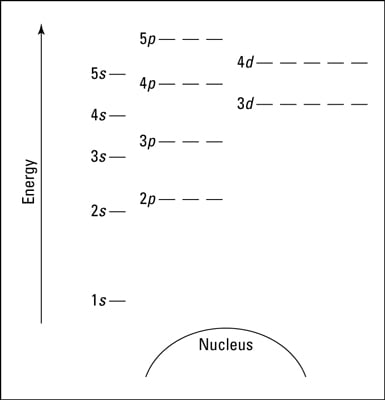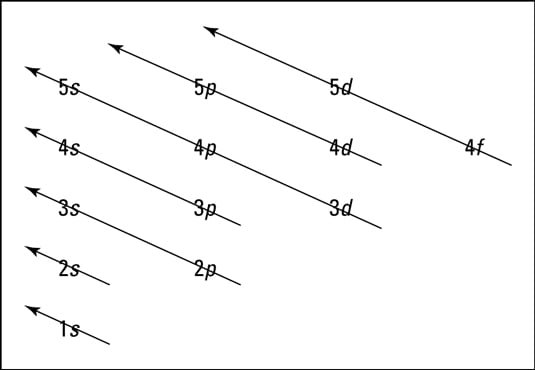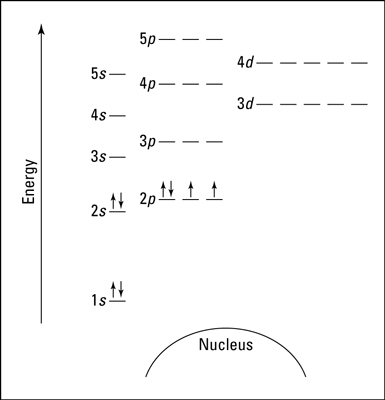##### Inorganic Chemistry For DummiesChemists sometimes use an energy level diagram to represent electrons when they’re looking at chemical reactions and bonding. An energy level diagram is more useful and easier to work with than quantum numbers in the quantum mechanical model.

Chemists use the energy level diagram as well as electron configuration notation to represent which energy level, subshell, and orbital are occupied by electrons in any particular atom. Chemists use this information in these ways:

• To predict what type of bonding will occur with a particular element and show exactly which electrons are being used

• To show why certain elements behave in similar ways

The following diagram is a blank energy level diagram you can use to depict electrons for any particular atom. Not all the known orbitals and subshells are shown. But with this diagram, you should be able to do most anything you need to.A blank energy level diagram.

In the preceding diagram, orbitals are represented with dashes in which you can place a maximum of two electrons. The 1s orbital is closest to the nucleus, and it has the lowest energy. It’s also the only orbital in energy level 1.

At energy level 2, there are both s and p orbitals, with the 2s having lower energy than the 2p. The three 2p subshells are represented by three dashes of the same energy.

Energy levels 3, 4, and 5 are also shown. Notice that the 4s has lower energy than the 3d: This is an exception to what you may have thought, but it’s what’s observed in nature.

The next diagram shows the Aufbau Principle, a method for remembering the order in which orbitals fill the vacant energy levels.The Aufbau Principle.
In using the energy level diagram, remember two things:
• Electrons fill the lowest vacant energy levels first.

• When there’s more than one subshell at a particular energy level, such as at the 3p or 4d levels, only one electron fills each subshell until each subshell has one electron. Then electrons start pairing up in each subshell. This rule is named Hund’s Rule.

Suppose you want to draw the energy level diagram of oxygen. You look on the periodic table and find that oxygen is atomic number 8. This number means that oxygen has 8 protons in its nucleus and 8 electrons. So you put 8 electrons into your energy level diagram.

You can represent electrons as arrows. If two electrons end up in the same orbital, one arrow faces up and the other faces down.

The first electron goes into the 1s orbital, filling the lowest energy level first, and the second one spin pairs with the first one.

Electrons 3 and 4 spin pair in the next lowest vacant orbital — the 2s.

Electron 5 goes into one of the 2p subshells (it doesn’t matter which one — they all have the same energy), and electrons 6 and 7 go into the other two totally vacant 2p orbitals.

The last electron spin pairs with one of the electrons in the 2p subshells (again, it doesn’t matter which one you pair it with). The completed energy level diagram for oxygen appears in the following illustration.An energy level diagram for oxygen.